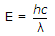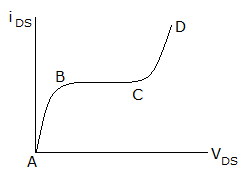Electronics and Communication Engineering - Electronic Devices and Circuits

31.

A zener diode is used in

 A. voltage regulator circuit B. amplifier circuits C. both voltage regulator and amplifier circuit D. none of the above

Explanation:

Zener diode is used only in voltage regulator circuits.

32.

A particular green LED emits light of wavelength 5490, Å, the energy bandgap of the semiconductor material used there is .. h = 6.6 x 10-34 J sec.

 A. 2.26 eV B. 1.98 eV C. 1.17 eV D. 0.74 eV

Explanation:

From Plank equationjoule

to convert it into electron volt it will be divided by 1.6 x 10-19.

33.

In a zener diode

 A. the forward current is very high B. sharp breakdown occurs at a certain reverse voltage C. the ratio v-i can be negative D. there are two p-n junctions

Explanation:

When reverse voltage equals breakdown value it starts conducting and voltage does not increase further.

34.

In a bipolar transistor which current is largest

 A. collector current B. base current C. emitter current D. base current or emitter current

Explanation:

Emitter current is larger, collector current is slightly less than emitter current and base current is very small.

35.

The v-i characteristics of a FET is shown in figure. In which region is the device biased for small signal amplificationA. AB B. BC C. CD D. BD